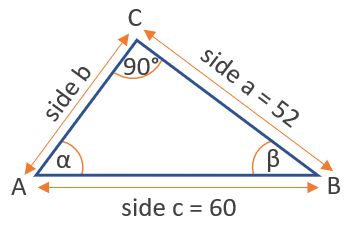# Arccos Calculator

Use this arccos calculator to easily calculate the arccosine of a number. Supports input of either decimal numbers (e.g. 0.5, -0.5) or fractions (e.g. 1/2, -1/2).

### Calculation results

60 degrees arccos(0.5) = arccos(0.5) =
Share calculator:

Embed this tool:
get code

## Arccos function

The arccos (arcus cosine, arccosine) is one of the inverse trigonometric functions (antitrigonometric functions, arcus functions) and is the inverse of the cosine function. It is sometimes written as cos-1(x), but this notation should be avoided as it can be confused with an exponent notation (power of, raised to the power of). The arccos is used to obtain an angle from the cosine trigonometric ratio, which is the ratio between the side adjacent to the angle and the hypotenuse in a right triangle.

The function spans from -1 to 1, and so do the results from our arccos calculator. The range of the angle values is usually between 0° and 180°. There are a number of arccos rules, like that cos(arccos(x)) = x, or that arccosα + arccosβ = arccos(αβ - √((1-α2)(1-β2)), as well as sine of the arccosine: sin(arccos(x)) = √(1-x2), which can help you in trigonometry calculus.

## How to calculate the arccosine of a number?

The easiest way to calculate it is by using our arccos calculator above, which will output results in both degrees and radians. Other ways include other given information, such as the values of other trigonometric functions for the same angle or other angles in the same triangle.

Here is a table of common arccos values:

Common values of the arccos function
-1 180° π
-√3 / 2 150° 5π/6
-√2 / 2 135° 3π/4
-1/2 120° 2π/3
0 90° π/2
1/2 60° π/3
√2 / 2 45° π/4
√3 / 2 30° π/5
1 π/6

π is, of course, the mathematical constant about equal to 3.14159.

## Example of using arccosine

Given a right angle triangle as in the figure below of which we know the length of side a = 52 and of the hypotenuse c = 60 and also knowing that the angle at point C is 90 degrees, find the angle β at point B using the inverse cosine function.By the definition of the cosine we know that the cosine of β equals the adjacent side divided by the hypotenuse: cos(β) = a / c = 52 / 60 = 0.866. Plugging this result in the inverse function we get that β = arccos(0.866) = 30° (or 0.52 in radians).

#### Cite this calculator & page

If you'd like to cite this online calculator resource and information as provided on the page, you can use the following citation:
Georgiev G.Z., "Arccos Calculator", [online] Available at: https://www.gigacalculator.com/calculators/arccos-calculator.php URL [Accessed Date: 01 Apr, 2023].